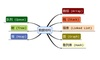• 数据结构实验哈希表Time Limit: 1000MS Memory Limit: 65536KB Submit Statistic Problem Description在n个数中，找出出现次数最多那个数字，并且输出出现的次数。如果有多个结果，输出数字最小的那一个。 ...
数据结构实验：哈希表
Time Limit: 1000MS Memory Limit: 65536KB  Submit Statistic  Problem Description
在n个数中，找出出现次数最多那个数字，并且输出出现的次数。如果有多个结果，输出数字最小的那一个。  Input
单组数据，第一行数字n（1<=n<=100000)。  接下来有n个数字，每个数字不超过100000000  Output
出现次数最多的数字和次数。  Example Input
3  1 1 2  Example Output
1 2  Hint
Author
cz

居然没超时………
#include <stdio.h>
#include <stdlib.h>
#include <string.h>
#define MAXN 100000000
int a[MAXN];
int main()
{
int n, b;
scanf("%d", &n);
memset(a, 0, sizeof(a));
for(int i=0;i<n;i++)
{
scanf("%d", &b);
a[b]++;
}
int point = 0;
int max = 0;
for(int i=0;i<MAXN;i++)
{
if(a[i]>max)
{
max = a[i];
point = i;
}
}
printf("%d %d\n", point ,max);
return 0;
}

哈希代码：
#include<stdio.h>
#include<string.h>

struct m
{
int data;
int tim;
struct m * next;
} a;

{
m *p = &a[n],*t;
t = p;
while (p != NULL)
{
if (p->data == d)
{
p->tim++;
return 0;
}
p = p->next;
if (t->next != NULL)
t = t->next;
}
p = t;
p->next = new m;
p = p->next;
p->data = d;
p->tim = 1;
p->next = NULL;
return 0;
}
int imax;
int fid (void)
{
int t = 0,n = 0,i;
m *p;
for (i = 0; i < 100000; i++)
{
p = &a[i];
while (p != NULL)
{
if (p->tim > t)
{
t = p->tim;
n = p->data;
}else if (p->tim == t)
{
if (p->data < n)
{
n = p->data;
}
}
p = p->next;
}
}
imax = n;
return t;
}

int main()
{
int n,i,m,t;
memset(a,0,sizeof (a));
scanf ("%d",&n);

for (i = 0; i < n; i++)
{
scanf ("%d",&t);
}
int num = fid();
printf ("%d %d\n",imax,num);
return 0;
}  
展开全文• SDUT 1480数据结构实验哈希表 Description 在n个数中，找出出现次数最多那个数字，并且输出出现的次数。如果有多个结果，输出数字最小的那一个。 Input 单组数据，第一行数字n（1<=n<=100000)。 接下来有n个...
SDUT 1480数据结构实验：哈希表
Description 在n个数中，找出出现次数最多那个数字，并且输出出现的次数。如果有多个结果，输出数字最小的那一个。 Input 单组数据，第一行数字n（1<=n<=100000)。 接下来有n个数字，每个数字不超过100000000 Output 出现次数最多的数字和次数。 Sample Input 3 1 1 2 Output 1 2
#include <iostream>
#include <cstdio>
#include <cstring>

using namespace std;

const int N = 100003, null = 0x3f3f3f3f;

int h[N], a[N], num[N];

int Find(int x)
{
int t = (x % N + N ) % N;
while(h[t] != null && h[t] != x)
{
t++;
if(t == N ) t = 0;
}
return t;
}

int main()
{
int n;
int j = 0;
scanf("%d",&n);

memset(h, 0x3f, sizeof(h));

while(n--)
{
int x;
scanf("%d",&x);
a[j++] = x;
h[Find(x)] = x;
num[Find(x)]++;
}

int maxnum = 0;
int k;
for(int i = 0 ; i < N ; i++)
{
int t = maxnum;
maxnum = max(maxnum, num[i]);
if(maxnum != t) k = i;
}

int res;
for(int i = 0 ; i < j ; i++)
{
if(Find(a[i]) == k)
{
res = a[i];
break;
}
}

printf("%d %d\n",res, maxnum);
return 0;
}

展开全文• 数据结构实验哈希表 Time Limit: 1000 ms Memory Limit: 65536 KiB Problem Description 在n个数中，找出出现次数最多那个数字，并且输出出现的次数。如果有多个结果，输出数字最小的那一个。 Input 单组数据，第...
数据结构实验：哈希表 Time Limit: 1000 ms Memory Limit: 65536 KiB
Problem Description 在n个数中，找出出现次数最多那个数字，并且输出出现的次数。如果有多个结果，输出数字最小的那一个。 Input 单组数据，第一行数字n（1<=n<=100000)。 接下来有n个数字，每个数字不超过100000000 Output 出现次数最多的数字和次数。 Sample Input 3 1 1 2 Sample Output 1 2
#include <stdio.h>
#include <stdlib.h>
#include <string.h>
int a;
int b;
int main()
{
int n,m,i,j,maxs=0,max=0;
memset(a,-1,sizeof(a));
memset(b,0,sizeof(b));
scanf("%d",&n);
m=n+1;
for(i=0; i<n; i++)  //输入n个数
{
int q;
int w;
scanf("%d",&q);
w=q%m;         //保留余数法
for(j=0; j<n; j++)     //如果不符合下面俩条件，说明这个位置被占用，j++，进行下一个位置的判断
{
if(a[w]==-1)
{
a[w]=q;
b[w]++;
if(maxs<b[w])
{
maxs=b[w];
max=a[w];
}
else if(maxs==b[w])
{
if(a[w]<max)
{
max=a[w];
}
}
break;
}
else if(a[w]==q)
{
b[w]++;
if(maxs<b[w])
{
maxs=b[w];
max=a[w];
}
else if(maxs==b[w])
{
if(a[w]<max)
{
max=a[w];
}
}
break;
}
}
}
printf("%d %d\n",max,maxs);
return 0;
}


展开全文算法 c语言
• 课题的目的和任务：根据数据元素的关键字和哈希函数建立哈希表并初始化哈希表，用开放定址法处理冲突，按屏幕输出的功能表选择所需的功能实现用哈希表数据元素的插入，显示，查找，删除。
• 数据结构实验哈希表 Time Limit: 1000 ms Memory Limit: 65536 KiB Problem Description 在n个数中，找出出现次数最多那个数字，并且输出出现的次数。如果有多个结果，输出数字最小的那一个。 Input 单组数据，第...
数据结构实验：哈希表 Time Limit: 1000 ms Memory Limit: 65536 KiB
Problem Description 在n个数中，找出出现次数最多那个数字，并且输出出现的次数。如果有多个结果，输出数字最小的那一个。 Input 单组数据，第一行数字n（1<=n<=100000)。 接下来有n个数字，每个数字不超过100000000 Output 出现次数最多的数字和次数。 Sample Input 3 1 1 2 Sample Output 1 2
#include <stdio.h>
#include <string.h>
#include <stdlib.h>
struct node
{
int data;
int num;
struct node *next;
}*h,*q,*t,*p;
int main()
{
int m,n;
int i,max=0,maxs=0;
for(i=0; i<100010; i++)
{
h[i]=(struct node*)malloc(sizeof(struct node)); //弄一个头结点
h[i]->next=NULL;
}
scanf("%d",&n);
for(i=0; i<n; i++)
{
scanf("%d",&m);
int w;
w=m%100010;          //取余
q=h[w];
p=h[w]->next;
while(p!=NULL)
{
if(p->data==m)
{
p->num++;
if(p->num>maxs)
{
maxs=p->num;
max=p->data;
}
else if(p->num==maxs)
{
if(p->data<max)
{
max=p->data;
}
}
break;      //如果有相等的直接跳出，并且下面那个if也不会执行
}
q=q->next;
p=p->next;
}
if(p==NULL)
{
t=(struct node*)malloc(sizeof(struct node));  //申请新的空间
t->next=NULL;
t->data=m;
t->num=1;    //在链表后面插入新的，置为1；
q->next=t;
p=t;
if(p->num>maxs)
{
maxs=p->num;
max=p->data;
}
else if(p->num==maxs)
{
if(p->data<max)
max=p->data;
}
}
}
printf("%d %d\n",max,maxs);
return 0;

}


展开全文链表 算法 c语言
• 数据结构》（C语言版本）的实验之一，哈希表设计。网上找的资源，共享。
• 数据结构实验哈希表 Time Limit: 1000ms Memory limit: 65536K有疑问？点这里^_^ 题目描述 在n个数中，找出出现次数最多那个数字，并且输出出现的次数。如果有多个结果，输出数字最小的那一个。 输入 单...
• 数据结构试验 哈希表的设计及实现 C语言 源代码
• 数据结构课程设计 哈希表实验报告 福建工程学院 课程设计 课程 算法与数据结构 题目 哈希表 专业 网络工程 班级 xxxxxx班 座号 xxxxxxxxxxxx 姓名: xxxxxxx 12月 31日 实验题目哈希表 一 要解决的问题 针对同班同学...
• 哈希表课程设计数据结构实验报告——哈希表设计 针对某个集体中的人名设计一个哈希表，使得平均查找长度不超过R，完成相应的建立和查表程序. 1.2 人名为汉语拼音形式，最长不超过18个字符(如：庄双双 ......

# 数据结构实验七哈希表数据结构 订阅